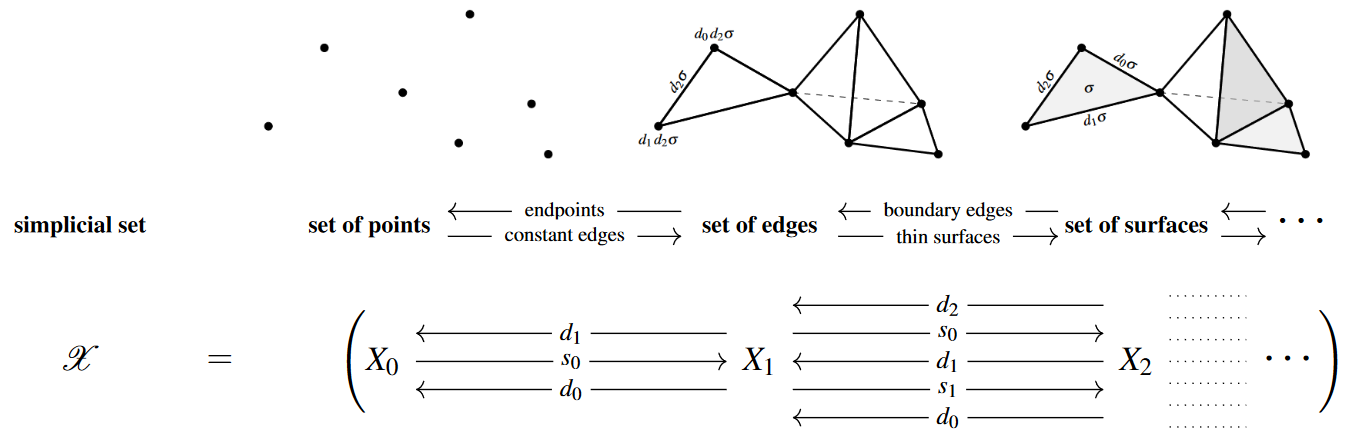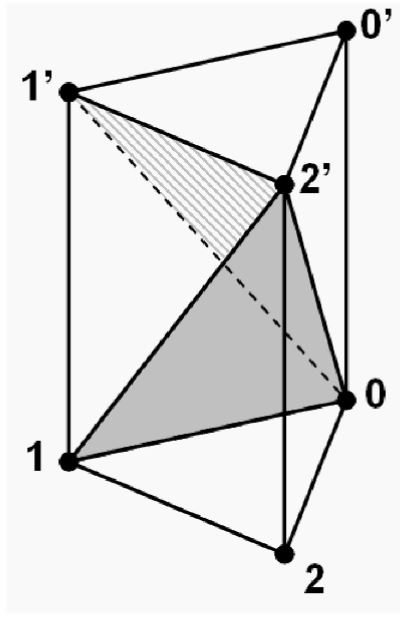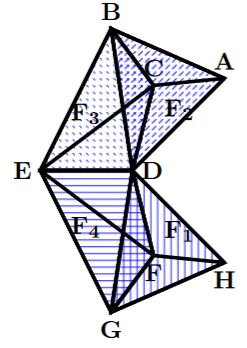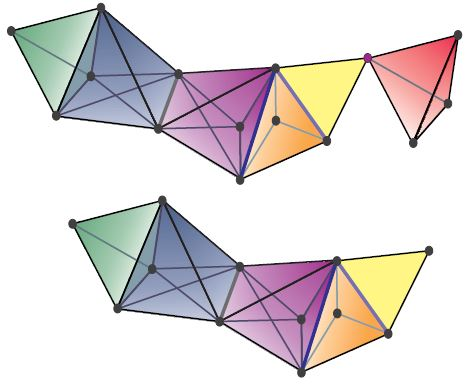# nLab simplicial set

Content

### Context

#### Combinatorics

combinatorics

enumerative combinatorics

graph theory

rewriting

Basic structures

Generating functions

Proof techniques

Combinatorial identities

Polytopes

category: combinatorics

# Content

## Idea

Simplicial sets generalize the idea of simplicial complexes: a simplicial set is like a combinatorial space built up out of gluing abstract simplices to each other. Equivalently, it is an object equipped with a rule for how to consistently map the objects of the simplex category into it.

More concretely, a simplicial set $S$ is a collection of sets $S_n$ for $n \in \mathbb{N}$, so that elements in $S_n$ are to be thought of as $n$-simplices, equipped with a rule that says:

• which $(n-1)$-simplices in $S_{n-1}$ are faces of which elements of $S_n$;
• which $(n+1)$-simplices are thin in that they are really just $n$-simplices regarded as degenerate $(n+1)$-simplices.

One of the main uses of simplicial sets is as combinatorial models for the (weak) homotopy type of topological spaces. They can also be taken as models for ∞-groupoids. This is encoded in the model structure on simplicial sets. For more reasons why simplicial sets see MathOverflow here.

## Definition

The quick abstract definition of a simplicial set goes as follows:

###### Definition

A simplicial set is a presheaf on the simplex category $\Delta$, that is, a functor $X : \Delta^{op} \to Sets$ from the opposite category of the simplex category to the category Set of sets; equivalently this a simplicial object in Set.

Equipped with the standard homomorphisms of presheaves as morphisms (namely natural transformations of the corresponding functors), simplicial sets form the category sSet (denoted both $SSet$ or $sSet$).

Explicitly this means the following.

###### Definition

(simplicial set)

A simplicial set $X \in sSet$ is

• for each $n \in \mathbb{N}$ a set $X_n \in Set$ – the set of $n$-simplices;

• for each injective map $\;\delta_i :\: [n-1] \to [n]\;$ of totally ordered sets ($[n] \coloneqq \{ 0 \lt 1 \lt \cdots \lt n \}$)

a function $\;d_i :\: X_{n} \to X_{n-1}\;$ – the $i$th face map on $n$-simplices ($n \gt 0$ and $0 \leq i \leq n$);

• for each surjective map $\;\sigma_i :\: [n+1] \to [n]\;$ of totally ordered sets

a function $\;s_i :\: X_{n} \to X_{n+1}\;$ – the $i$th degeneracy map on $n$-simplices ($n \geq 0$ and $0 \leq i \leq n$);

such that these functions satisfy the simplicial identities.

$\,$## Remarks

### Simplicial sets as spaces built of simplices

• The definition is to be understood from the point of view of space and quantity: a simplicial set is a space characterized by the fact that it may be probed by mapping standard simplices into it: the set $S_n$ assigned by a simplicial set to the standard $n$-simplex $[n]$ is the set of $n$-simplices in this space, hence the way of mapping a standard $n$-simplex into this space.

• For $S$ a simplicial set, the face map

$d_i \coloneqq S(\delta^i): S_n \rightarrow S_{n-1}$

is dual to the unique injection $\delta^i : [n-1] \rightarrow [n]$ in the category $\Delta$ whose image omits the element $i \in [n]$.

• Similarly, the degeneracy map

$s_i \coloneqq S(\sigma^i) : S_n \rightarrow S_{n+1}$

is dual to the unique surjection $\sigma^i : [n+1] \rightarrow [n]$ in $\Delta$ such that $i \in [n]$ has two elements in its preimage.

• The maps $\delta^i$ and $\sigma^i$ satisfy certain obvious relations – the simplicial identities – dual to those spelled out at simplex category.

### Visualisation

(based on cubical set)

The face maps go from sets $S_{n+1}$ of $(n+1)$-dimensional simplices to the corresponding set $S_{n}$ of $n$-dimensional simplices and can be thought of as sending each simplex in the simplicial set to one of its faces, for instance for $n=1$ the set $S_2$ of 2-simplices would be sent in three different ways by three different face maps to the set of $1$-simplices, for instance one of the face maps would send

$\left( \array{ & & b \\ & \nearrow & \Downarrow^F & \searrow \\ a & & \rightarrow & & c } \right) \;\; \mapsto \;\; \left( \array{ & & b \\ & \nearrow \\ a } \right)$

another one would send

$\left( \array{ & & b \\ & \nearrow & \Downarrow^F & \searrow \\ a & & \rightarrow & & c } \right) \;\; \mapsto \;\; \left( \array{ a & & \rightarrow & & c } \right) \,.$

On the other hand, the degeneracy maps go the other way round and send sets $S_n$ of $n$-simplices to sets $S_{n+1}$ of $(n+1)$-simplices by regarding an $n$-simplex as a degenerate or “thin” $(n+1)$-simplex in the various different ways that this is possible. For instance, again for $n=1$, a degeneracy map may act by sending

$\left( \array{ a &\stackrel{f}{\to}& b } \right) \;\; \mapsto \;\; \left( \array{ & & b \\ & \nearrow_f & \Downarrow^{Id} & \searrow^{Id} \\ a & & \stackrel{f}\to & & b } \right) \,.$

Notice the $Id$-labels, which indicate that the edges and faces labeled by them are “thin” in much the same way as an identity morphism is thin. They depend on lower dimensional features, (notice however that a simplicial set by itself is not equipped with any notion of composition of simplices, nor really, therefore, of identities. See quasicategory for a kind of simplicial set which does have such notions and simplicial T-complex for more on the intuitions behind this idea of “thinness”).

## Examples

### The empty simplicial set

The empty simplicial set is a simplicial set.

### $n$-simplices (Yoneda embeddings)

Let $[n]$ denote the object of the simplex category $\Delta$ corresponding to the totally ordered set $\{ 0, 1, 2,\ldots, n\}$. Then the represented presheaf $\Delta(-, [n])$, typically written as $\Delta[n]$ is an example of a simplicial set. In particular we have $\Delta[n]_m=Hom_\Delta([m],[n])$ and hence $\Delta[n]_m$ is a finite set with $\binom{n+m+1}{n}$ elements.

By the Yoneda lemma, the $n$-simplices of a simplicial set $X$ are in natural bijective correspondence to maps $\Delta[n] \rightarrow X$ of simplicial sets.

### The minimal simplicial circle

See at minimal simplicial circle.

### Cartesian products of simplices

The non-degenerate simplices in the Cartesian product of simplices

$\Delta \times \Delta$

of the 1-simplex $\Delta$ with the 2-simplex $\Delta$ (i.e. the canonical simplicial cylinder object over the 2-simplex) is the simplicial set which looks as follows:graphics grabbed from Friedman 08, p. 33

For more on this and related examples see at product of simplices.

### Simplicial complexes

Every simplicial complex can be viewed a simplicial set (in several different ways).graphics grabbed form arXiv:1710.06129graphics grabbed from Maletic, 2013

In particular any graph is thought of as being built of vertices and edges and so is a (1-dimensional) simplicial complex. Choosing a direction on the edges then gives a directed graph and that gives a simplicial set, as follows.

### Directed graphs

A directed graph (with loops and multiple edges allowed, i.e., a quiver) $E \rightrightarrows V$ is essentially the same thing as a 1-dimensional simplicial set, by taking $S_0 \coloneqq V$ to be the set of vertices and $S_1 \coloneqq E \uplus V$ to be the disjoint union of the set of edges with the set of vertices (the latter corresponding to the degenerate 1-simplices).

### Nerve of a category

If $C$ is a small category, the nerve of $C$ is a simplicial set which we denote $NC$. If we intepret the poset $[n]$ defined above as a category, we define the $n$-simplices of $NC$ to be the set of functors $[n] \rightarrow C$. Equivalently, the $0$-simplices of $NC$ are the objects of $C$, the $1$-simplices are the morphisms, and the $n$-simplices are strings of $n$ composable arrows in $C$. Face maps are given by composition (or omission, in the case of $d_0$ and $d_n$) and degeneracy maps are given by inserting identity arrows.

### Singular simplicial complex of a topological space

Recall from simplex category or geometric realization the standard functor $\Delta \to Top$ which sends $[n] \in \Delta$ to the standard topological $n$-simplex $\Delta^n$. This functor induces for every topological space $X$ the simplicial set

$S X : [n] \mapsto Hom_{Top}(\Delta^n, X)$

called the simplicial singular complex of $X$. This simplicial set is always a Kan complex and may be regarded as the fundamental ∞-groupoid of $X$.

Following up on the idea of ‘’thinness’’, a singular simplex $f: \Delta^n \to X$ may be called thin if it factors through a retraction $r: \Delta^n \to \Lambda^{n-1}_i$ to some horn of $\Delta^n$, then the well known Kan condition on $S X$ can be strengthened to say that every horn in $S X$ has a thin filler. This also helps to give some intuitive underpinning to the idea of thin element in this simplicial context.

### Bar construction

For the moment see bar construction.

## Properties

### Basic properties

###### Proposition

(simplicial set is colimit of its elements)
Every simplicial set $X \in sSet$ is the colimit of its simplices, in that

\begin{aligned} X & \;\simeq\; \underset{ \Delta[k] \in el(X) }{lim} \Delta[k] \\ & \;\simeq\; \int^{[k] \in \Delta} \Delta[k] \times X_k \end{aligned}

Here the first line shows a colimit over the category of elements of $X$ (e.g. Goerss & Jardine, I, Lemma 2.1) and the second line shows the corresponding coend-expression (by this Prop.).
###### Proof

Since SimplicialSets is a category of presheaves (over the simplex category $\Delta$) this is a special case of the general fact that every presheaf is a colimit of representables (this Prop., the “co-Yoneda lemma”).

###### Remark

In fact, the colimit in Prop. is a homotopy colimit, see there.

### Classifying topos

#### Simplicial sets

The category of simplicial sets is a presheaf category, and so in particular a Grothendieck topos. In fact, it is the classifying topos of the theory of “intervals”, meaning totally ordered sets equipped with distinct top and bottom elements.

Specifically, if $E$ is a topos containing such an interval $I$, then we obtain a functor $\Delta \to E$ sending $[n]$ to the subobject

$\{ (x_1,x_2,\dots,x_n) \;|\; x_1 \le x_2 \le \dots \le x_n \} \hookrightarrow I^n$

The corresponding geometric realization/nerve adjunction $E \leftrightarrows Set^{\Delta^{op}}$ is the geometric morphism which classifies $I$. In particular, the generic such interval is the simplicial 1-simplex $\Delta^1$; see generic interval for more.

The usual geometric realization into topological spaces cannot be obtained in this way precisely, since Top is not a topos. However, there are Top-like categories which are toposes, such as Johnstone's topological topos.

#### Cosimplicial sets

Similarly, also the category $Set^{\Delta}$ of cosimplicial sets is a classifying topos: for inhabited linear orders. See at classifying topos the section For (inhabited) linear orders.

### As models in homotopy theory

(…) homotopy theory (…) Kan complex (…) quasi-category (…)

### Relation to dendroidal sets

For the moment see at dendroidal set the section Relation to simplicial sets

## Variants

• A symmetric set is a simplicial set equipped with additional transposition maps $t^n_i: X_n \to X_n$ for $i=0,\ldots,n-1$. These transition maps generate an action of the symmetric group on $X_n$ and satisfy certain commutation relations with the face and degeneracy maps.

The original definition:

Further early discussions (aimed at simplicial homotopy theory):

Exposition and introduction:

Further discussion in the context of simplicial homotopy theory/algebraic topology:

category: topology